×
Get Full Access to University Physics - 13 Edition - Chapter 26 - Problem 28e
Get Full Access to University Physics - 13 Edition - Chapter 26 - Problem 28e

×

# In the circuit shown in Fig. E26.28, find (a) the currentISBN: 9780321675460 31

## Solution for problem 28E Chapter 26

University Physics | 13th Edition

• Textbook Solutions
• 2901 Step-by-step solutions solved by professors and subject experts
• Get 24/7 help from StudySoup virtual teaching assistantsUniversity Physics | 13th Edition

4 5 1 433 Reviews
20
0
Problem 28E

In the circuit shown in ?Fig. E26.28?, find (a) the current in each branch and (b) the potential difference ?V?ab? ?of point ?a ?relative to point ?b?.

Step-by-Step Solution:

Problem 28E

In the circuit shown in Fig. E26.28, find (a) the current in each branch and (b) the potential difference Vab of point a relative to point b.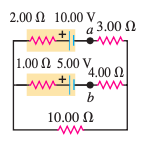Step By Step Solution:

Step 1 of 3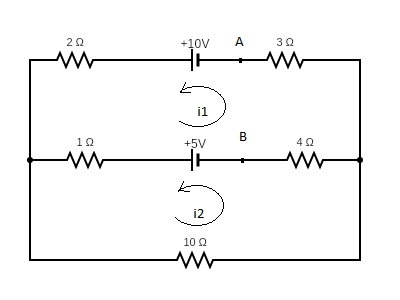We’re taking the current in the upper loop as i1 and the lower loop as i2.

Applying Kirchoffs voltage law in the upper loop that is the sum of voltage around a closed loop is zero.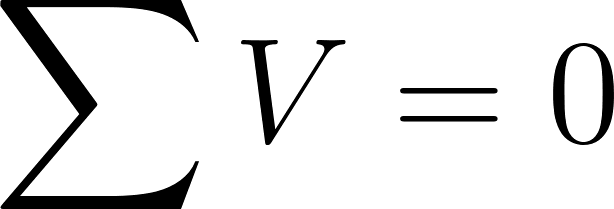ie.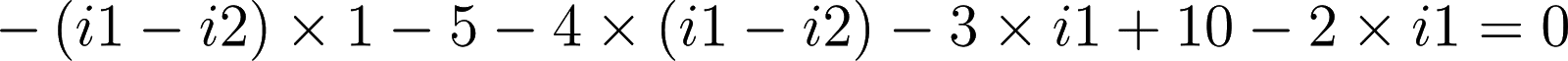ie.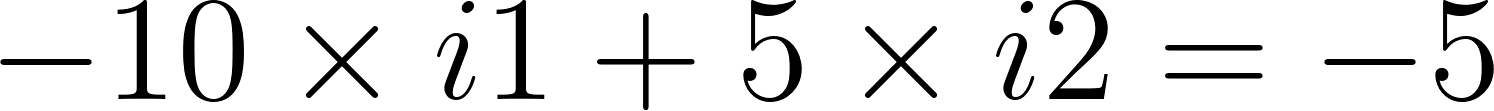ie.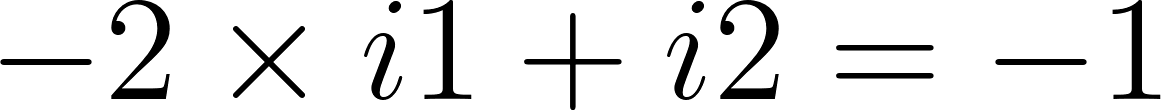ie.(1)

Step 2 of 3

Step 3 of 3

##### ISBN: 9780321675460

This textbook survival guide was created for the textbook: University Physics, edition: 13. This full solution covers the following key subjects: point, Find, current, difference, fig. This expansive textbook survival guide covers 26 chapters, and 2929 solutions. University Physics was written by and is associated to the ISBN: 9780321675460. The full step-by-step solution to problem: 28E from chapter: 26 was answered by , our top Physics solution expert on 05/06/17, 06:07PM. Since the solution to 28E from 26 chapter was answered, more than 692 students have viewed the full step-by-step answer. The answer to “In the circuit shown in ?Fig. E26.28?, find (a) the current in each branch and (b) the potential difference ?V?ab? ?of point ?a ?relative to point ?b?.” is broken down into a number of easy to follow steps, and 27 words.

Unlock Textbook Solution